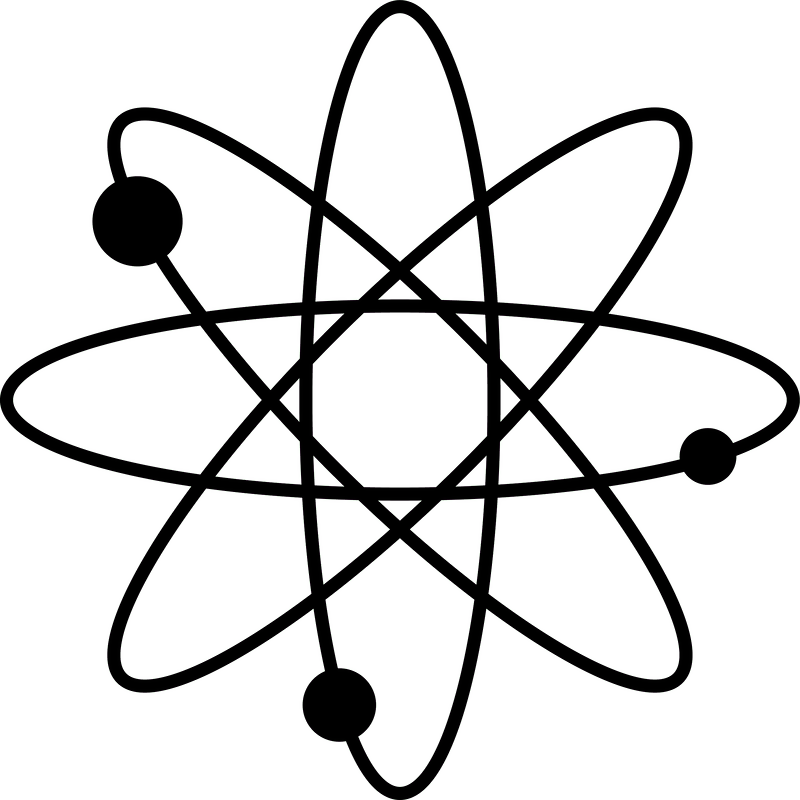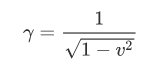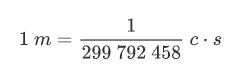###Unit Analysis

We are used from Newtonian mechanics tothink about distances, time intervals and velocities in terms of units likemph, m, km, s, m/s etc. However, in special relativity the scale where therelativistic phenomena become visible is very different. Because this scale isdetermined by the speed of light, it would greatly simplify our calculationsand reasoning if we were to employ a system of units adapted to this constant.

Distances

The speed of light in the vacuum isapproximately

c300 000 000 m/s

This is an inconveniently long number. Weeither need a larger unit for distance, or a smaller unit of time. For manyreasons, the second seems to be a more adequate unit of time. Instead, we canintroduce a new unit of distance, defined as the distance covered by light inone second.

We'll call this the light−second:

1 light−second=1 cs300 000 000 m

Notice that the light-second is a unit fora dimension of distance.

Using our new unit, we can re-write c as:

c=1 light−second/second

Velocities

It is a consequence of special relativitythat there are no velocities greater than the speed of light. This makes thespeed of light into a natural scale for measuring velocities. You have alreadyseen that γ was defined in terms of v/c. This suggests that it would be auseful simplification to express all velocities in units of c. For example,v=0.1 c instead of writing v=30 000 000 m/s.

Then we would re-write γ as:where v is measured in units of c.

The utility of these units will become muchclearer in Lecture 4.

Natural Units

In our previous discussion of dimensionlessanalysis, we have tried to motivate the choice of time and distance asfundamental dimensions. At the same time, because the speed of light is auniversal constant, it could suggest us that we can also express all thedistances in terms of the speed of light and some time unit. For example, ameter could be defined asSo now our two yardsticks are c and s. Butthere is a fundamental difference between them: c has a well defined physicalmeaning, while s still needs to be defined in terms of some arbitrary numericalvalue. A second is defined as the "the duration of 9 192 631 770 periodsof the radiation corresponding to the transition between the two hyperfinelevels of the ground state of the caesium 133 atom" (SI Brochure, Section2.1.1.3). Because we wanted to preserve the historical value of the second, wehad to introduce that messy number.

Because the speed of light is free of anyarbitrary values, it's called a natural unit. You should be careful, becausewhen using natural units some physicists like to write c=1, but this is not areal equality, but a misnomer. c is a dimensionful quantity ([c]=L/T) while 1is dimensionless.

We will continue our discussion of naturalunits in the future lessons. Our goal is to show how we can employ otherfundamental constants to develop a system of natural units.